﻿ 基于数据空间和稀疏约束的三维重力和重力梯度数据联合反演
 地球物理学报2021, Vol. 64Issue (3): 1074-1089PDF

1. 吉林大学地球探测科学与技术学院, 长春 130026;
2. 中国地质调查局广州海洋地质调查局, 广州 510760;
3. 长春工程学院, 长春 130021

Three-dimensional joint inversion of gravity and gravity gradient data based on data space and sparse constraints
ZHANG RongZhe1, LI TongLin1, LIU Cai1, LI FuYuan2, DENG XinHui3, SHI HuiYan1
1. College of Geo-Exploration Sciences and Technology, Jilin University, Changchun 130026, China;
2. Guangzhou Marine Geological Survey, China Geological Survey, Guangzhou 510760, China;
3. Changchun Institute of Technology, Changchun 130021, China
Keywords: Gravity data    Gravity gradient data    Conjugate gradient method    Sparse constraints    Data space    Joint inversion
0 引言

1 快速正演计算理论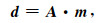(1)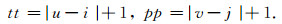(2)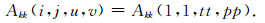(3)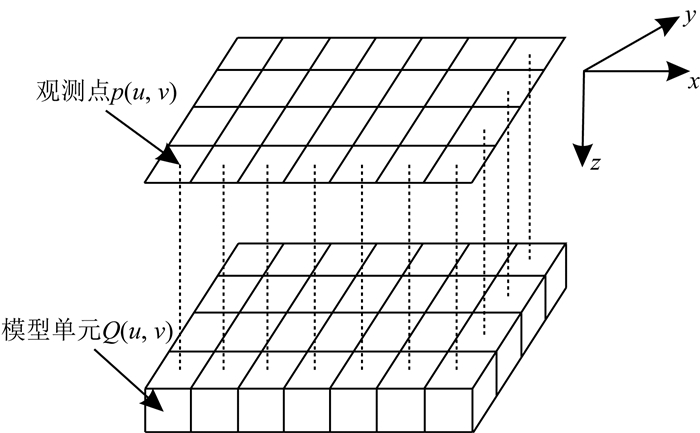图 1 三维模型网格剖分图 Fig. 1 3D model meshing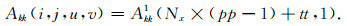(4)

if ui,

Akk(i, j, u, v)=-Akk1(Nx×(pp-1)+tt, 1)

else Akk(i, j, u, v)=Akk1(Nx×(pp-1)+tt, 1)

end

if vj,

Akk(i, j, u, v)=-Akk1(Nx×(pp-1)+tt, 1)

else Akk(i, j, u, v)=Akk1(Nx×(pp-1)+tt, 1)

end

if uivj

if ui && vj,

Akk(i, j, k, l)=-Akk1(Nx×(pp-1)+tt, 1)

else Akk(i, j, u, v)=-Akk1(Nx×(pp-1)+tt, 1)

end

else Akk(i, j, u, v)=Akk1(Nx×(pp-1)+tt, 1)

end

2 反演计算理论 2.1 目标函数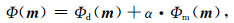(5)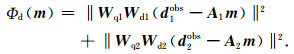(6)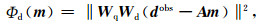(7)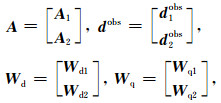(8)

L0范数正则化模型约束项的表达式如下：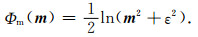(9)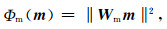(10)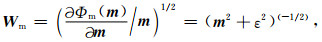(11)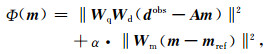(12)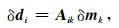(13)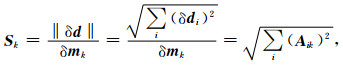(14)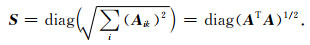(15)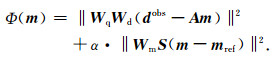(16)

2.2 目标函数的优化

2.2.1 传统共轭梯度法反演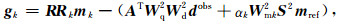(17)

While kk_max或misfit＜σ

k=k+1；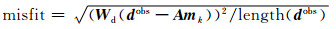end While

2.2.2 改进的共轭梯度法反演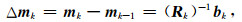(18)

While kk_max或misfit＞σ(外部循环)

x0=0, r0=d0=bk

While ii_max或sqrt(riTri)＞10-6(内部循环)

i=i+1；

ri=ri-1-ti-1Rkdi-1

end While

k=k+1；

mk=mk-1+xi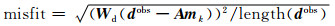end While

2.3 数据空间共轭梯度法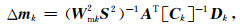(19)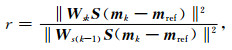(20)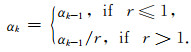(21)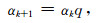(22)

3 模型试算 3.1 单独和联合反演对比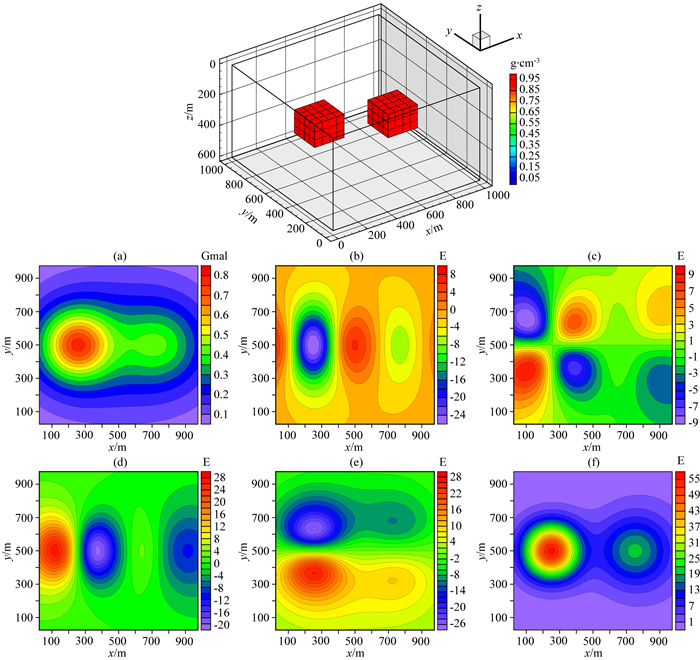图 2 模型一的理论模型和正演响应等值线图 (a) 重力gz; (b) 重力梯度分量Vxx; (c) 重力梯度分量Vxy; (d) 重力梯度分量Vzx; (e) 重力梯度分量Vzy; (f) 重力梯度分量Vzz. Fig. 2 Theoretical model and forward response contour map of model 1 (a) Gravity gz; (b) Gravity gradient component Vxx; (c) Gravity gradient component Vxy; (d) Gravity gradient component Vzx; (e) Gravity gradient component Vzy; (f) Gravity gradient component Vzz.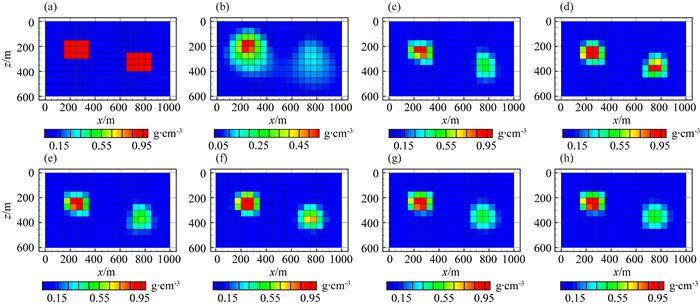图 3 不同观测数据反演结果对比图 (a) 理论模型; (b) gz反演结果; (c) gz和Vxx联合反演结果; (d) gz和Vxy联合反演结果; (e) gz和Vzx联合反演结果; (f) gz和Vzy联合反演结果; (g) gz和Vzz联合反演结果; (h) gz和全张量联合反演结果; 垂向切片位于y=550 m处. Fig. 3 Comparison of different observation data inversion results (a) Theoretical model; (b) gz inversion results; (c) gz and Vxx joint inversion results; (d) gz and Vxy joint inversion results; (e) gz and Vzx joint inversion results; (f) gz and Vzy joint inversion results; (g) gz and Vzz joint inversion results; (h) gz and full tensor joint inversion results; The cross section is located at y=550 m.表 1 反演模型和理论模型的均方根误差 Table 1 The root mean square error of each inversion model and the theoretical model
3.2 传统和改进的共轭梯度法反演对比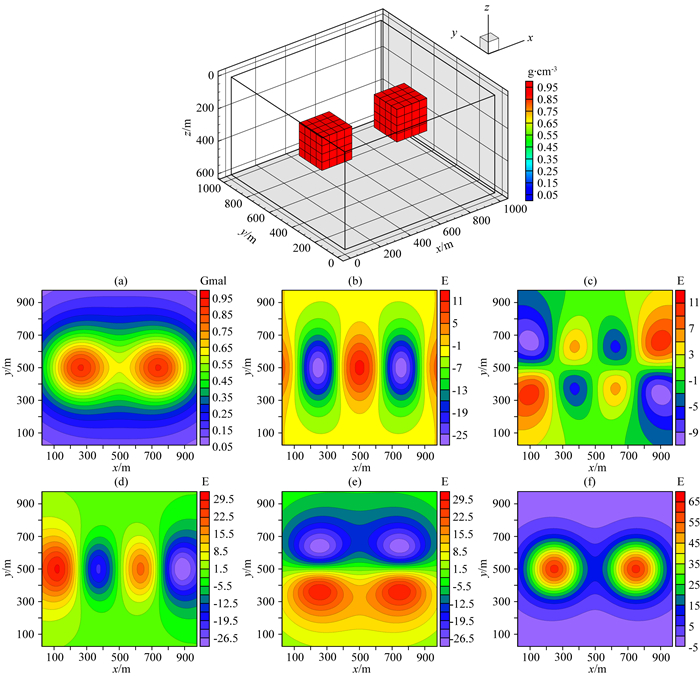图 4 模型二的理论模型和正演响应等值线图 (a) 重力gz; (b) 重力梯度分量Vxx; (c) 重力梯度分量Vxy; (d) 重力梯度分量Vzx; (e) 重力梯度分量Vzy; (f) 重力梯度分量Vzz. Fig. 4 Theoretical model and forward response contour map of model 2 (a) Gravity gz; (b) Gravity gradient component Vxx; (c) Gravity gradient component Vxy; (d) Gravity gradient component Vzx; (e) Gravity gradient component Vzy; (f) Gravity gradient component Vzz.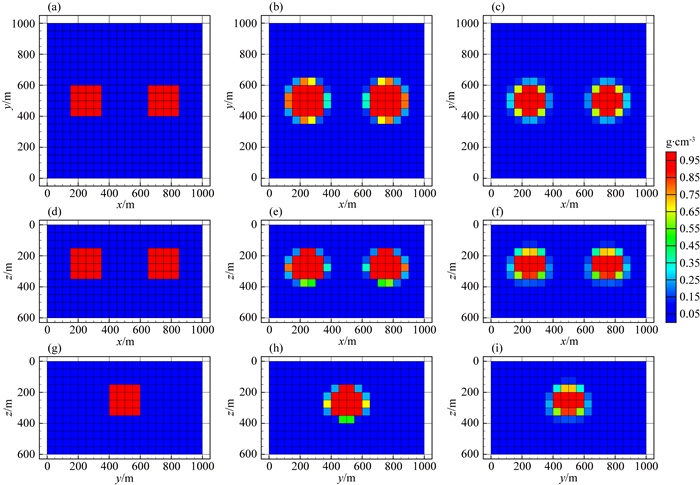图 5 传统和改进的共轭梯度法反演结果对比图 (a, d, g) 理论模型; (b, e, h) 传统的共轭梯度法反演结果; (c, f, i) 改进的共轭梯度法反演结果; (a, b, c) z=300 m处的横向切片; (d, e, f) y=500 m处的垂向切片; (g, h, i) x=250 m处的垂向切片图. Fig. 5 Comparison of traditional and improved conjugate gradient inversion results (a, d, g) Theoretical model; (b, e, h) Traditional conjugate gradient inversion results; (c, f, i) Improved conjugate gradient inversion results; (a, b, c) In the z=300 m depth section; (d, e, f) In the y=500 m cross section; (g, h, i) In the x=250 m cross section.
3.3 模型积分灵敏度矩阵和深度加权矩阵对比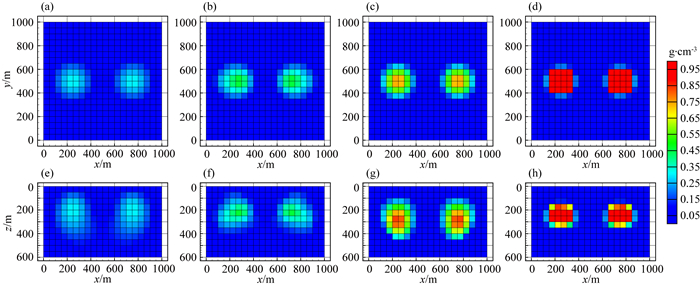图 6 不同S矩阵的平滑和稀疏约束反演结果的对比图 (a, b, c, d) z=250 m处的横向切片; (e, f, g, h) y=500 m处的垂向切片; (a, e) 深度加权平滑反演结果; (b, f) 深度加权稀疏约束反演结果; (c, g) 模型积分灵敏度平滑反演结果; (d, h) 模型积分灵敏度稀疏约束反演结果. Fig. 6 Comparison of smooth and sparse constraint inversion results of different S matrices (a, b, c, d) In the z=250 m depth section; (e, f, g, h) In the y=500 m cross section; (a, e) The depth weighted smooth inversion results; (b, f) The depth weighted sparse inversion results; (c, g) The model integral sensitivity smooth inversion results; (d, h) The model integral sensitivity sparse inversion results.
3.4 模型空间和数据空间法对比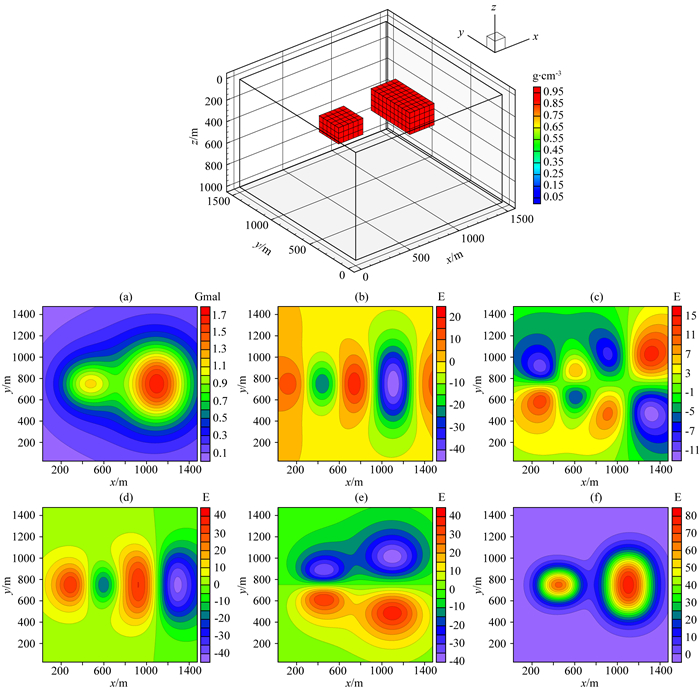图 7 模型三的理论模型和正演响应等值线图 (a) 重力gz; (b) 重力梯度分量Vxx; (c) 重力梯度分量Vxy; (d) 重力梯度分量Vzx; (e) 重力梯度分量Vzy; (f) 重力梯度分量Vzz. Fig. 7 Theoretical model and forward response contour map of model 3 (a) Gravity gz; (b) Gravity gradient component Vxx; (c) Gravity gradient component Vxy; (d) Gravity gradient component Vzx; (e) Gravity gradient component Vzy; (f) Gravity gradient component Vzz.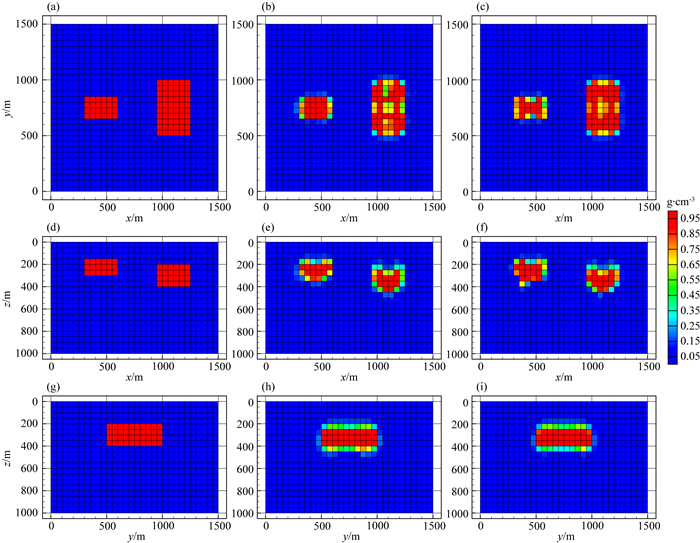图 8 模型空间和数据空间联合反演结果对比图 (a, d, g) 理论模型; (b, e, h) 模型空间法反演结果; (c, f, i) 数据空间法反演结果; (a, b, c) z=300 m处的横向切片; (d, e, f) y=750 m处的垂向切片; (g, h, i) x=1150 m处的垂向切片图. Fig. 8 Comparison of model space and data space joint inversion results (a, d, g) Theoretical model; (b, e, h) The model space inversion results; (c, f, i) The data space inversion results; (a, b, c) In the z=300 m depth section; (d, e, f) In the y=750 m cross section; (g, h, i) In the x=1150 m cross section.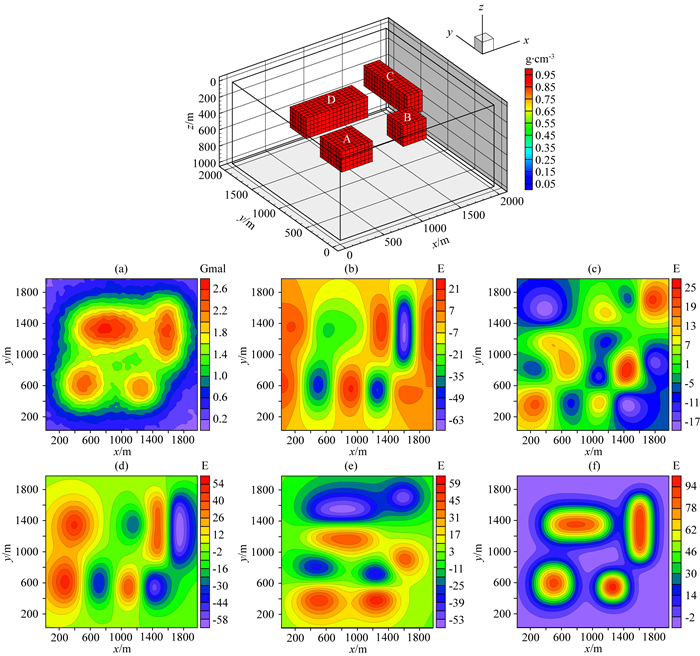图 9 模型四的理论模型和正演响应等值线图 (a) 重力gz; (b) 重力梯度分量Vxx; (c) 重力梯度分量Vxy; (d) 重力梯度分量Vzx; (e) 重力梯度分量Vzy; (f) 重力梯度分量Vzz. Fig. 9 Theoretical model and forward response contour map of model 4 (a) Gravity gz; (b) Gravity gradient component Vxx; (c) Gravity gradient component Vxy; (d) Gravity gradient component Vzx; (e) Gravity gradient component Vzy; (f) Gravity gradient component Vzz.表 2 理论模型异常体属性情况 Table 2 Theoretical model anomaly attributes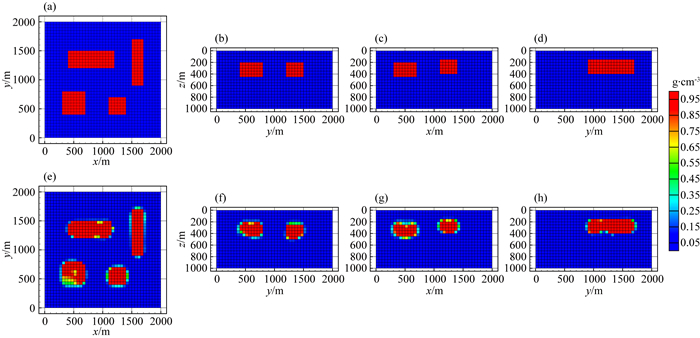图 10 数据空间共轭梯度法联合反演结果图 (a, b, c, d) 理论模型; (e, f, g, h) 联合反演结果; (a, e) z=300 m处的横向切片; (b, f) x=600 m处的垂向切片; (c, g) y=550 m处的垂向切片; (d, h) x=1600 m处的垂向切片. Fig. 10 The data space conjugate gradient method joint inversion results (a, b, c, d) Theoretical model; (e, f, g, h) Joint inversion results; (a, e) In the z=300 m depth section; (b, f) In the x=600 m cross section; (c, g) In the y=550 m cross section; (d, h) In the x=1600 m cross section.
4 实测数据应用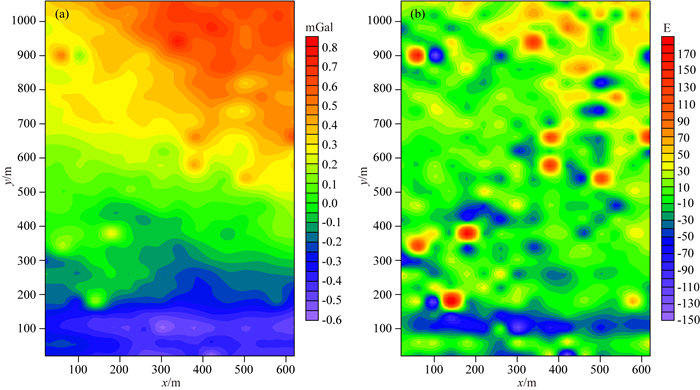图 11 实测重力和重力梯度数据等值线图 (a) 重力数据; (b) 重力梯度数据Vzz. Fig. 11 Contour plots of measured gravity and gravity gradient data (a) The gravity data; (b)The gravity gradient data Vzz.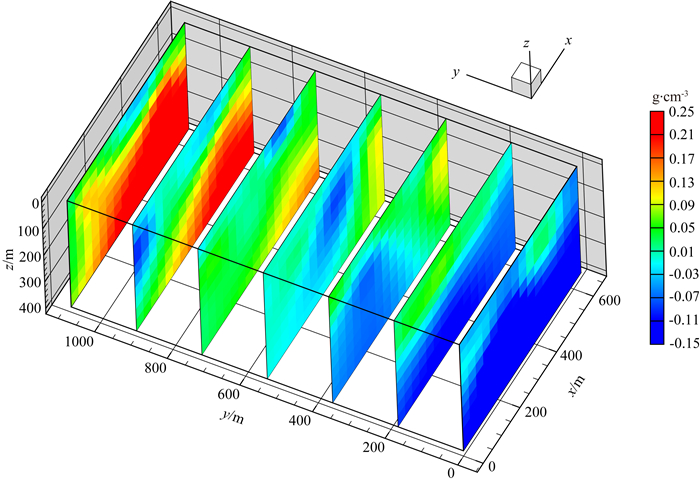图 12 重力和重力梯度数据联合反演获得的密度分布垂向切片图 Fig. 12 Cross section of density distribution obtained by joint inversion of gravity and gravity gradient data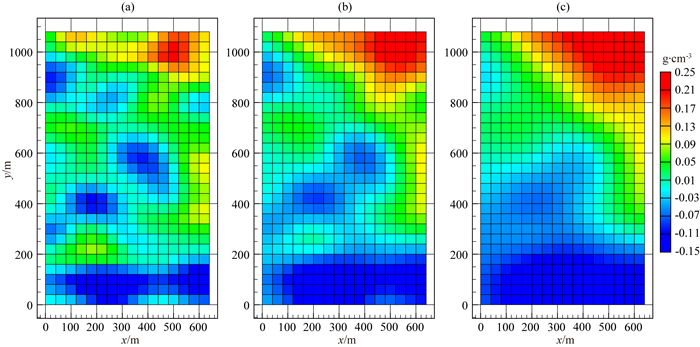图 13 重力和重力梯度数据联合反演获得的密度分布横向切片图 (a) z=100 m处的横向切片; (b) z=200 m处的横向切片; (c) z=300 m处的横向切片. Fig. 13 Depth section of density distribution obtained by joint inversion of gravity and gravity gradient data (a) In the z=100 m depth section; (b) In the z=200 m depth section; (c) In the z=300 m depth section.
5 结论

References